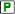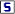# Generate random numbers¶has a number of distribution functions included, in order for you to be able to generate random numbers very efficiently. This capability might be extremely useful when you want to quickly populate a multidimensional parameter.

Say I declare a parametercalled Traffic, over 2indices from the same setLocations with a size of 200 elements. That’s pretty much 200 * 200 = 40 000 numbers that you want to generate roughly between 0 and 200 vehicles.

Let’s create a setLocations in AIMMS, specify 2 indices l1 and l2, and define it as elementrange(1,201,1,"Location-").

Then let’s create the new parameter that we want to populate with data, over those 2 indices. To do so, just index this parameter over l1 and l2, and put uniform(0,200) in his definition. Check the data of this parameter ( CTRL + D ), and see the result:

If we would plot our parameter distribution we would end up with a uniform distribution.proposes a lot of other different distributions, listed here

See the AIMMS example project GenerateRandomNumbers.zip.

Normal Distribution

$$f(x)={\tfrac {1}{\sigma {\sqrt {2\pi }}}}\;\;{\mathrm {e}}^{{-{\frac {1}{2}}\left({\frac {x-\mu }{\sigma }}\right)^{2}}}$$

https://en.wikipedia.org/wiki/Log-normal_distribution

Log Normal Distribution

$$f(x;\mu ,\sigma )={\frac {1}{x\sigma {\sqrt {2\pi }}}}\exp \left(-{\frac {(\ln x-\mu )^{2}}{2\sigma ^{2}}}\right)={\frac {1}{x}}f_{X}(\ln(x);\mu ,\sigma )$$

https://en.wikipedia.org/wiki/Log-normal_distribution

Last Updated: November, 2019